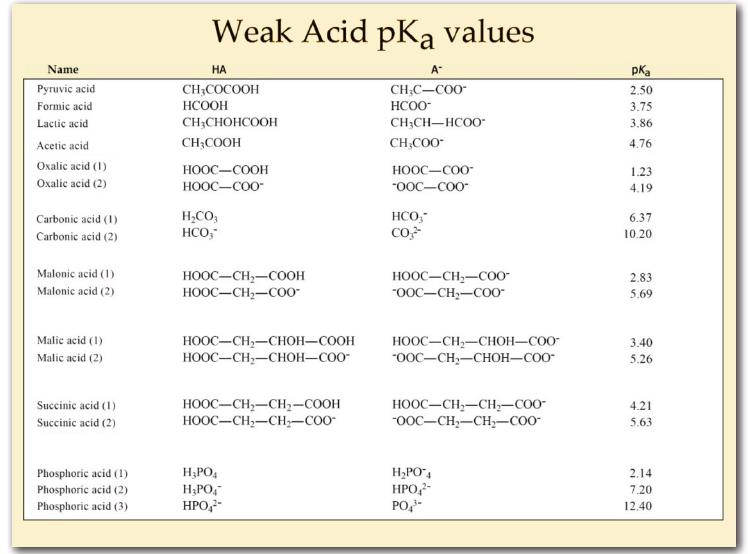# 1.4: Buffers Keep the Cellular Environment Stable

Water can ionize to a slight extent ($$10^{-7}\; M$$ is about 6 molecules per 100 million of pure water) to form $$H^+$$ (proton) and $$OH^-$$ (hydroxide). We measure the proton concentration of a solution with pH, which we define as the negative log of the proton concentration.

$pH = -\log[H^+] \label{1.4.1}$

If the proton concentration, $$[H^+]= 10^{-7}\; M$$, then the pH is 7. We could just as easily measure the hydroxide concentration with the pOH by the parallel equation, $$pOH = -\log[OH^-]$$ In pure water, dissociation of a proton from it creates a hydroxide, so the pOH of pure water is 7, as well. This also means that

$pH + pOH = 14 \label{1.4.2}$

Now, because protons and hydroxides can combine to form water, a large amount of one will cause there to be a small amount of the other. Why is this the case? In simple terms, if I dump 0.1 moles of $$H^+$$ into a pure water solution, the high proton concentration will react with the relatively small amount of hydroxides to create water, thus reducing hydroxides. Similarly, if I dump excess hydroxide (as $$NaOH$$, for example) into pure water, the proton concentration falls for the same reason.Figure 1.4.1: Chart of pKa values for weak acids.

Chemists use the term “acid” to refer to a substance which has protons that can dissociate (come off) when dissolved in water. They use the term “base” to refer to a substance that can absorb protons when dissolved in water. Both acids and bases come in strong and weak forms. Strong acids, such as HCl, dissociate completely in water. If we add 0.1 moles of $$HCl$$ to a solution to make a liter, it will have 0.1 moles of $$H^+$$ and 0.1 moles of Cl-. There will be no remaining $$HCl$$ when this happens. A strong base like NaOH also dissociates completely into $$Na^+$$ and $$OH^-$$.

Weak acids and bases differ from their strong counterparts. When you put one mole of acetic acid (HAc) into pure water, only about 4 in 1000 HAc molecules dissociate into $$H^+$$ and $$Ac^-$$. Thus, if I start with 1000 $$HAc$$, I will end up with 996 $$HAc$$ and 4 each of $$H^+$$ and $$Ac^-$$.

Clearly, weak acids are very different from strong acids. Weak bases behave similarly, except that they accept protons, rather than donate them.

You may wonder why we care about weak acids.You may never have thought much of weak acids when you were in General Chemistry. Your instructor described them as buffers and you probably dutifully memorized the fact that “buffers are substances that resist change in pH” without really learning what it meant. We will not allow that to happen here.

Weak acids are critical for life because their affinity for protons causes them to behave like a UPS. We’re not referring to the UPS that is the United Parcel Service®, but instead, to the encased battery backup systems for computers called Uninterruptible Power Supplies that kick on to keep a computer running during a power failure. Your laptop battery is a UPS, for example. We can think of weak acids as Uninterruptible Proton Suppliers within certain pH ranges, providing (or absorbing) protons as needed.Weak acids thus help to keep the H+ concentration (and thus the pH) of the solution they are in relatively constant.

Consider the acetic acid (acetate) system. Here is what happens when $$HAc$$ dissociates

$HAc \rightleftharpoons H^+ + Ac^- \label{1.4.3}$

As noted, about 4 in 1000 $$HAc$$ molecules come apart. However, what if one started adding hydroxyl ions (by adding a strong base like $$NaOH$$) to the solution with the $$HAc$$ in it? As the added $$OH$$ ions reacted with the $$H^+$$ ions to make water, the concentration of $$H^+$$ ions would go down and the pH would go up. However, in contrast to the situation with a solution of pure water, there is a backup source of $$H^+$$ available in the form of $$HAc$$. Here is where the UPS function kicks in. As protons are taken away by the added hydroxyl ions (making water), they are partly replaced by protons from the $$HAc$$. This is why a weak acid is a buffer. It resists changes in pH by releasing protons to compensate for those “used up” in reacting with the hydroxyl ions.Figure 1.4.2: An example buffer system - carbonic acid/bicarbonate. Note that near pH 6.73, addition of $$OH^-$$ results in only small pH changes. This is what a buffer does - resists changes in pH over certain ranges.